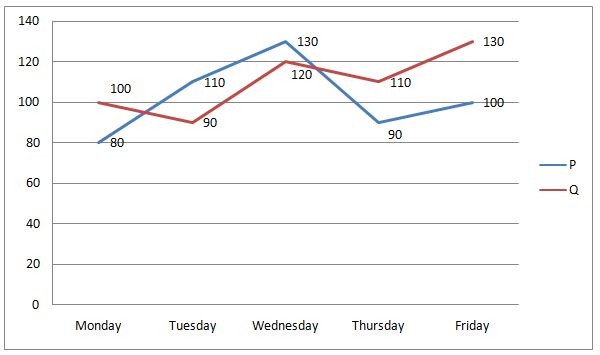# IBPS SO / NIACL AO Prelims – Quantitative Aptitude Questions Day- 65

Dear Readers, Bank Exam Race for the Year 2018 is already started, To enrich your preparation here we have providing new series of Practice Questions on Quantitative Aptitude – Section. Candidates those who are preparing for IBPS SO / NIACL AO Prelims 2018 Exams can practice these questions daily and make your preparation effective.

[WpProQuiz 4734]

Directions (Q. 1 – 5): What value should come in place of question mark (?) in the following questions?

1) [(1088 ÷ 17)1/2 + (396 ÷ 11)2] = (?)2 + 79

a) 38

b) 42

c) 35

d) 33

e) None of these

2) 25 % of 1200 ÷ 15 + 12 % of 250 =? – 40 % of 850

a) 390

b) 440

c) 280

d) 520

e) None of these

3) 20 % of ? – 6859 ÷ 19 – 375 ÷ 3 = 86

a) 1350

b) 2180

c) 1840

d) 2860

e) None of these

4) (4/9) of 252 + 322 ÷ 8 × 4 + 162 =? × 4

a) 290

b) 220

c) 350

d) 470

e) None of these

5) ? × 5 = (569 + 781) ÷ 9 + 232 + (3/7) of 840 – 239

a) 280

b) 120

c) 160

d) 340

e) None of these

Directions (Q. 6 – 10): Study the following information carefully and answer the given questions.

The following line graph shows the total kilocalories burned by 2 individuals (P and Q) on treadmill in 5 different days in a certain week.6) If the total kilocalories burned by P and Q are increased by 10 % and 20 % respectively from Friday to Saturday, then find the ratio between the total kilocalories burned by P in Wednesday and Saturday together to that of total kilocalories burned by Q in Wednesday and Saturday together?

a) 20: 23

b) 15: 17

c) 6: 11

d) 35: 43

e) None of these

7) Find the difference between the total kilocalories burned by P to that of Q in all the given days together?

a) 60

b) 90

c) 50

d) 40

e) None of these

8) Total number of kilocalories burned by P in Monday, Tuesday and Friday together is approximately what percentage more/less than the total kilocalories burned by Q in Wednesday, Thursday and Friday together?

a) 20 % more

b) 20 % less

c) 15 % less

d) 30 % more

e) 30 % less

9) If the average kilocalories burned by P in Monday, Wednesday, Friday and Saturday together is 115, then find the total kilocalories burned by P in Saturday?

a) 150

b) 140

c) 125

d) 135

e) None of these

10) Total kilocalories burned by P in Monday and Tuesday together is approximately what percentage of total kilocalories burned by Q in Wednesday?

a) 145 %

b) 125 %

c) 160 %

d) 110 %

e) 175 %

[(1088 / 17)1/2 + (396 / 11)2] = x2 + 79

(64)1/2 + (36)2 – 79 = x2

8 + 1296 – 79 = x2

X2 = 1225

X = 35

25 % of 1200 ÷ 15 + 12 % of 250 = x – 40 % of 850

(25/100)*(1200/15) + (12/100)*250 = x – (40/100)*850

20 + 30 + 340 = x

X = 390

20 % of x – 6859 ÷ 19 – 375 ÷ 3 = 86

(20/100)*x – (6859/19) – (375/3) = 86

(x/5) – 361 – 125 = 86

(x/5) = 86 + 361 + 125

X = 572*5 = 2860

(4/9) of 252 + 322 ÷ 8 × 4 + 162 = 4x

(4/9)*252 + (32*32*4)/8 + 256 = 4x

112 + 512 + 256 = 4x

880/4 = x

X = 220

5x = (569 + 781) ÷ 9 + 232 + (3/7) of 840 – 239

5x = (1350/9) + 529 + (3/7)*840 – 239

5x = 150 + 529 + 360 – 239

5x = 800

X = 160

The total kilocalories burned by P in Saturday

= > 100*(110/100) = 110

The total kilocalories burned by Q in Saturday

= > 130*(120/100) = 156

The total kilocalories burned by P in Wednesday and Saturday together

= > 130 + 110 = 240

The total kilocalories burned by Q in Wednesday and Saturday together

= > 120 + 156 = 276

Required ratio = 240: 276 = 20: 23

The total kilocalories burned by P in all the given days together

= > 80 + 110 + 130 + 90 + 100 = 510

The total kilocalories burned by Q in all the given days together

= > 100 + 90 + 120 + 110 + 130 = 550

Required difference = 550 – 510 = 40

Total kilocalories burned by P in Monday, Tuesday and Friday together

= > 80 + 110 + 100 = 290

The total kilocalories burned by Q in Wednesday, Thursday and Friday together

= > 120 + 110 + 130 = 360

Required % = [(360 – 290)/360]*100 = 19.44 % = 20 % less

The average kilocalories burned by P in Monday, Wednesday, Friday and Saturday together = 115

The total kilocalories burned by P in Monday, Wednesday, Friday and Saturday together = 115*4 = 460

The total kilocalories burned by P in Saturday

= > 460 – (80 + 130 + 100)

= > 460 – 310 = 150

Total kilocalories burned by P in Monday and Tuesday together

= > 80 + 110 = 190

Total kilocalories burned by Q in Wednesday = 120

Required % = (190/120)*100 = 158.33 % = 160 %